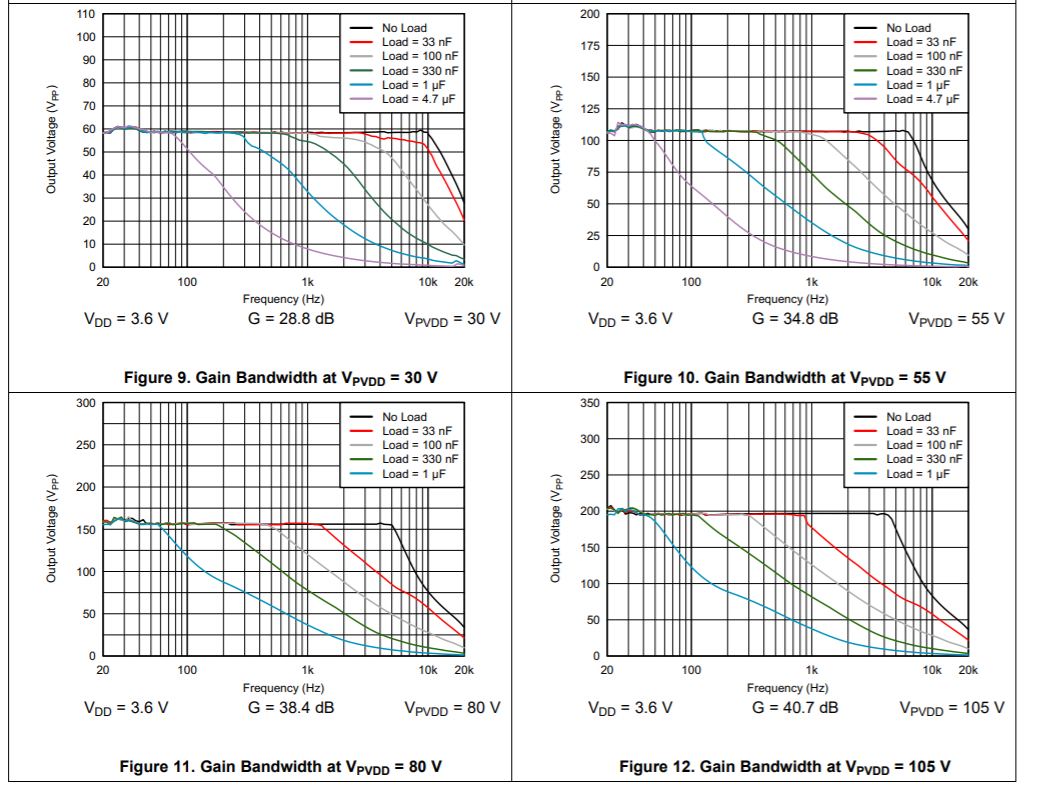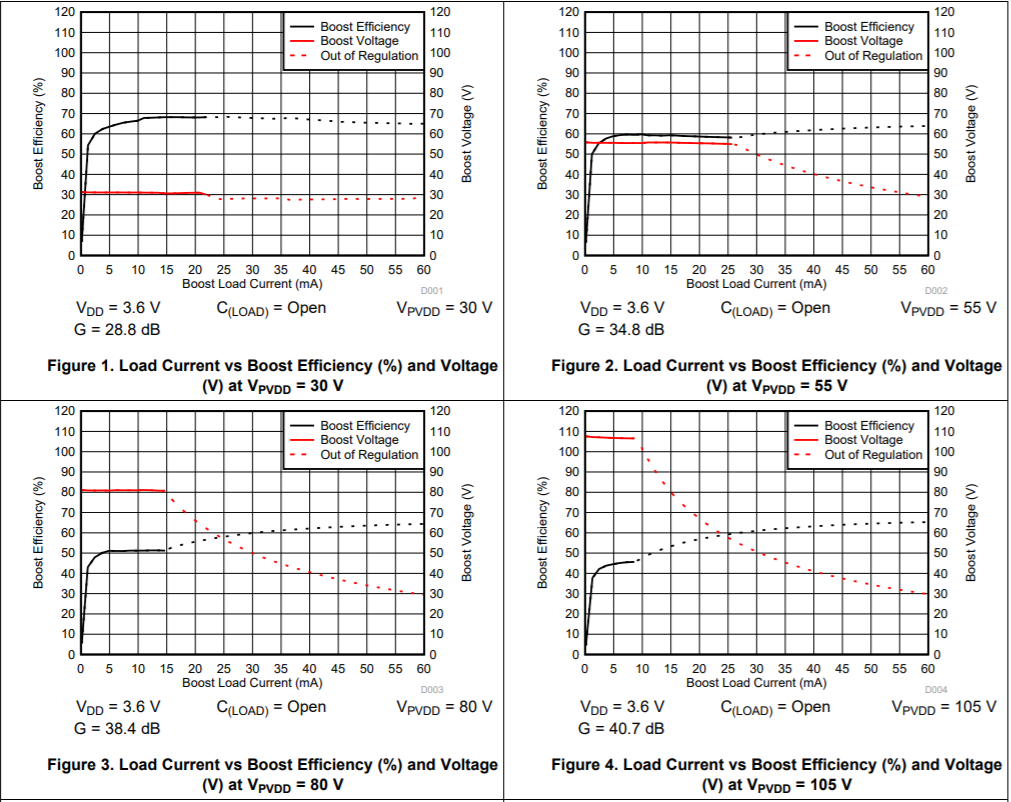If you have a related question, please click the "Ask a related question" button in the top right corner. The newly created question will be automatically linked to this question.

• Resolved

# [FAQ] DRV2700: Frequency Response

Part Number: DRV2700

DRV2700 Frequency Response

Being able to understand how the relationship between bandwidth, load capacitance, gain, and current limit is critical for getting the output you would expect when using the DRV2700

Looking at these graphs taken from the datasheet, we can see in each graph as we increase capacitance the bandwidth drops. If you have a piezo with capacitance not listed, you can easily interpolate in between the values to understand what you should expect. When we go across graphs and the gain increases the bandwidth continues to drop for each capacitance value. For example looking at the 28.8 dB gain chart a load of 4.7 uF is already has a incredibly low bandwidth and by the time you reach maximum gain the 4.7 uF load can’t even be supported.This relationship between variables is important in understanding that there is a limit to the amount of gain that can obtained with one load at high frequencies, so trying to increase the gain on a 30nF load to achieve higher voltages at higher frequencies will not work because bandwidth will decrease to the point to that you now have even less gain than before you tried to raise it.

So when trying to increase gain, it is more practical to change frequencies or load capacitance to achieve a working result.

Furthermore, not shown in these graphs is how the current limit affects gain. Increasing the current limit at a given load and gain will actually increase the cutoff frequencies in the order of kHz. Current limit is of course augmented through the Rext resistors.

But of course increasing the current too much will start drawing down boost efficiency which would then drive down your gain, as shown by the these graphs.